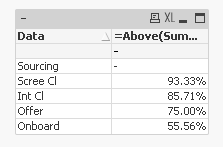# New to QlikView

Discussion board where members can get started with QlikView.

Announcements
Save the Date: QlikWorld Online, June 24-25, 2020. Free global virtual event for data integration and data analytic gurus. Register Today
New Contributor

## Sum of values below divided by sum of values one cell above and below

Hi,

I have an excel file which has data like below

 Data Cleared Status % Calc Sourcing 10 0% Scree Cl 20 0.933333 Int Cl 30 0.857143 Offer 40 0.75 Onboard 50

% calc is field I need to derive in qlikview.

The way it is calculated as is

(20+30+40+50)/(10+20+30+40+50)=0.933.. similarly (30+40+50)/(20+30+40+50)=0.8571.

I tried different combination of Rangesum function but it didn't work.

Tags (3)
1 Solution

Accepted Solutions
HighlightedMVP

## Re: Sum of values below divided by sum of values one cell above and below

May be this

=Above(Sum(TOTAL [Cleared Status]) - RangeSum(Above(Sum([Cleared Status]), 0, RowNo())))/Above(Sum(TOTAL [Cleared Status]) - RangeSum(Above(Sum([Cleared Status]), 1, RowNo())))2 Replies
HighlightedMVP

## Re: Sum of values below divided by sum of values one cell above and below

May be this

=Above(Sum(TOTAL [Cleared Status]) - RangeSum(Above(Sum([Cleared Status]), 0, RowNo())))/Above(Sum(TOTAL [Cleared Status]) - RangeSum(Above(Sum([Cleared Status]), 1, RowNo())))Highlighted
New Contributor

Perfect..Thanks